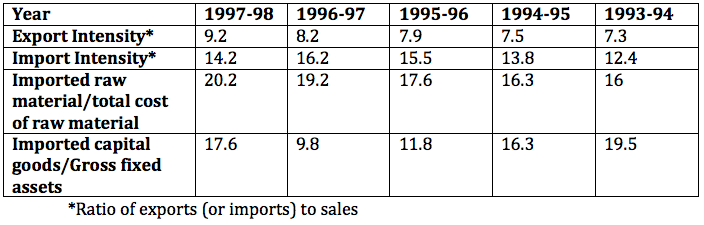### CAT 2000 Question Paper Question 24

Instructions

Directions for the next 4 questions: Answer these questions based on the table below:

The table shows trends in external transactions of Indian corporate sector during the period 1993-94 to 1997-98. In addition, following definitions hold good:

Sales, Imports, and Exports, respectively denote the sales, imports and exports in year i.

Deficit in year I, Deficit1 = Imports - Exports

Deficit Intensity in year I, DI = Deficit/Sales Growth rate of deficit intensity in year I, GDI = $$\frac{DI_i - DI_{i-1}}{DI_{i-1}}$$

Further, note that all imports are classified as either raw material or capital goods.

Trends in External Transactions of Indian Corporate Sector (All figures in %)Question 24

# In 1997-98 the total cost of raw materials is estimated as 50% of sales of that year. The turn over of Gross fixed assets, defined as the ratio of sales to Gross fixed assets, in 1997-98 is, approximately;

Solution

We know that Import / slaes = 14.2 and total import = 20.2*cost of raw material + 17.6 * gross fixed assets = 20.2*0.5*sales +17.6*GFA = 14.2* sales . Solving this we get 17.6 * GFA = 4.1 * sales . So required value is 17.6/4.1 = 4.3 . Hence option B .